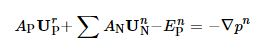# 关于icoFoam的一个小问题

• 我在看‘icoFoam解析’这篇文章，在推导方程的时候有一个小问题， 为什么公式 （14） 中的压力没有在体积和时间上积分啊？ 前面的一些推导对瞬态项，对流项，扩散项都进行了体积分和时间积分，但在公式（14）中压力梯度直接用了。谢谢！

• @mengweilm425
那个写得很详细是为了体现离散的过程。回归问题本身，最后就是要推导出来压力泊松方程。考虑下面简单的方程：
\begin{equation}
\nabla \cdot \mathbf{U} \mathbf{U} = - \nabla p
\end{equation}
即：
\begin{equation}
\mathbf{U} = f \left( \nabla p \right)
\end{equation}
结合连续性方程就有：
\begin{equation}
\nabla \cdot \left( f \left( \nabla p \right) \right) = 0
\end{equation}
这个看起来简单点。通常书上都是这个简单的写法。

另外，半离散方程就是慢慢来离散。先离散速度，压力不管。等推导出压力泊松方程之后。一起离散laplacian那一项。

• 非常感谢您的解答，我再好好推一推，有问题再跟您交流！

• @cfd-china $-\nabla p$ 应该写成 -fvc::grad(p) 更不容易引起误解，因为方程左边是对单元体积分并用高斯定理和离散后得到的矩阵，而右边是没有对单元体进行积分的。